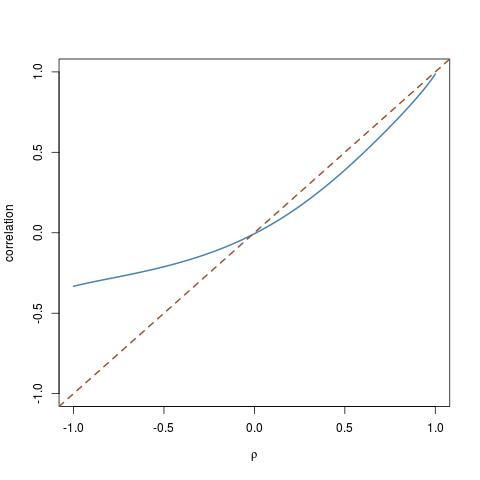arbitrary distributions with set correlationA question recently posted on X Validated by Antoni Parrelada: given two arbitrary cdfs F and G, how can we simulate a pair (X,Y) with marginals  F and G, and with set correlation ρ? The answer posted by Antoni Parrelada was to reproduce the Gaussian copula solution: produce (X’,Y’) as a Gaussian bivariate vector with correlation ρ and then turn it into (X,Y)=(F⁻¹(Φ(X’)),G⁻¹(Φ(Y’))). Unfortunately, this does not work, because the correlation does not keep under the double transform. The graph above is part of my answer for a χ² and a log-Normal cdf for F amd G: while corr(X’,Y’)=ρ, corr(X,Y) drifts quite a  lot from the diagonal! Actually, by playing long enough with my function

tacor=function(rho=0,nsim=1e4,fx=qnorm,fy=qnorm)
{
x1=rnorm(nsim);x2=rnorm(nsim)
coeur=rho
rho2=sqrt(1-rho^2)
for (t in 1:length(rho)){
y=pnorm(cbind(x1,rho[t]*x1+rho2[t]*x2))
coeur[t]=cor(fx(y[,1]),fy(y[,2]))}
return(coeur)
}

Playing further, I managed to get an almost flat correlation graph for the admittedly convoluted call

tacor(seq(-1,1,.01),
fx=function(x) qchisq(x^59,df=.01),
fy=function(x) qlogis(x^59))Now, the most interesting question is how to produce correlated simulations. A pedestrian way is to start with a copula, e.g. the above Gaussian copula, and to twist the correlation coefficient ρ of the copula until the desired correlation is attained for the transformed pair. That is, to draw the above curve and invert it. (Note that, as clearly exhibited by the graph just above, all desired correlations cannot be achieved for arbitrary cdfs F and G.) This is however very pedestrian and I wonder whether or not there is a generic and somewhat automated solution…

15 Responses to “arbitrary distributions with set correlation”

1.Quartz Says:

Well I guess a mixture of independence and perfect dependence copulae is obvious and a bit pathological, but it should solve the issue relatively easily when the marginals lead to a nice invertible parametrization of correlation. From there the path to something smoother might be clearer at least.

2.Clara Says:

The paper http://www.ualberta.ca/~csproat/Actuarial/Members/Extra%20Information/Copulas.pdf
summarizes a group of algorithms to simulate outcomes from a multivariate distribution (mostly proposed by Lee or Genest) based on the concept of generator of a copula and the inverse transform method.
One interesting point is that, at least to the best of my knowledge, there is no method to simulate from a multivariate (not only bivariate) distribution by fixing the multivariate correlation (like the multivariate rho).

•xi'an Says:

Thanks, Clara. However the paper states that “Pearson correlation coefficient depends not only on the copula but also on the marginal distributions. Thus, this measure is affected by (nonlinear) changes of scale.” Which just states an impossibility theorem in terms of achieving a given correlation through copulas.

•Clara Says:

But if this is certainly the case for the Pearson correlation coefficient, this is not true for others correlation measures as the Kendall’s coefficient.

3.Kees Mulder Says:

There is also an R package that attempts to do this through permutation:

http://cran.r-project.org/web/packages/correlate/index.html

•xi'an Says:

Interesting, Kees: I just tested it with 10000 simulations and my R program crashed. Will try again!

•xi'an Says:

Another attempt and now it does not crash. And it works indeed! Great!

•Kees Mulder Says:

I think crashing is not unlikely with this sort of approach: the dataset is reordered repeatedly in order to move towards the desired correlation. However, the values in `data` are never changed, only reordered, so getting a very high correlation on a small dataset will not get convergence.

So, correlate(replicate(2, rnorm(10)), .96) will probably crash. For many cases where we do not require an exact or extreme correlation in the output dataset, however, it might be a nice approach.

•xi'an Says:

I feel more and more queasy about this approach! It aims at an empirical correlation, rather than an actual correlation…

•Kees Mulder Says:

That’s definitely true. It’s more of an approximate, hacky solution, rather than a nice, exact, mathematical solution.

4.Daniele Says:
•xi'an Says:

Thanks. From this blog, I understand there is a linear way to transform a data matrix into another one with the desired correlation matrix. However the linear transform does not preserve the marginals when, e.g., those marginals are Gamma or t distributions.

5.Wolfgang Betz Says:

If I am not mistaken, the answer you are looking for can be found in:

(it is the Nataf distribution):

In particular eq.(11) in:
A. Der Kiureghian, P.-L. Liu, Structural reliability under incomplete probability information, J. Eng. Mech. ASCE 112 (1) (1986) 85–104.

Ditlevsen: structural reliability methods (chapter 7.2):
http://od-website.dk/books/OD-HOM-StrucRelMeth-Ed2.3.7.pdf
(see also Appendix 2 for some common approximate solutions)

•xi'an Says:

Thanks for the suggestion. Reading from this book, it seems that the Nataf distribution is a specific distribution. Which does not help with the quest for a generic algorithm for arbitrary marginals and fixed correlation.

•Wolfgang Betz Says:

It is based on Gaussian copula and works for arbitrary marginals and fixed correlations. However, not for all possible combinations there exists a solution; e.g., for some marginals there are restrictions on the correlations that can be represented. However, in practice this is usually not an issue, as this tends to happen when the target correlation approaches -1 or 1.

This site uses Akismet to reduce spam. Learn how your comment data is processed.